R packages:

# Install necessary packages
list_packages <- c("forecast", "readxl", "stargazer", "fpp",
"fpp2", "scales", "quantmod", "urca",
"vars", "tseries", "ggplot2", "dplyr")
new_packages <- list_packages[!(list_packages %in% installed.packages()[,"Package"])]
if(length(new_packages)) install.packages(new_packages)

lapply(list_packages, require, character.only = TRUE)

# 1 Box-Jenkins Methodology and ARIMA Models

Note that Box is George Box who said “all models are wrong, some are useful”.

There is a class of parametric time series models, autoregressive integrated moving average (ARIMA) models, which provides a rational basis for the generating mechanism of time series data. To work with ARIMA for prediction, we will follow the 3-stage iterative procedure as follows.

1. identification: selection of tentative ARIMA model using sample ACF $$\hat{\rho}_k$$.

Example: Business Inventories We analyzed the quarterly change in bupiness inventories, stated at annual rates in billions of dollars. We examine 60 observations covering the period from the first quarter of 1955 through the fourth quarter of 1969. The data is seasonally adjusted.

Q: Think about what could be the model? AR(1), AR(2), ARMA(1,1), ARMA(3,4)?

case1=as.ts(scan("case1.txt"))
case1
## Time Series:
## Start = 1
## End = 60
## Frequency = 1
##    4.4  5.8  6.7  7.1  5.7  4.1  4.6  4.3  2.0  2.2  3.6 -2.2 -5.1 -4.9  0.1
##   4.1  3.8  9.9  0.0  6.5 10.8  4.1  2.7 -2.9 -2.9  1.5  5.7  5.0  7.9  6.8
##   7.1  4.1  5.5  5.1  8.0  5.6  4.5  6.1  6.7  6.1 10.6  8.6 11.6  7.6 10.9
##  14.6 14.5 17.4 11.7  5.8 11.5 11.7  5.0 10.0  8.9  7.1  8.3 10.2 13.3  6.2
par(mfrow=c(1,3))
plot(case1); acf(case1); pacf(case1)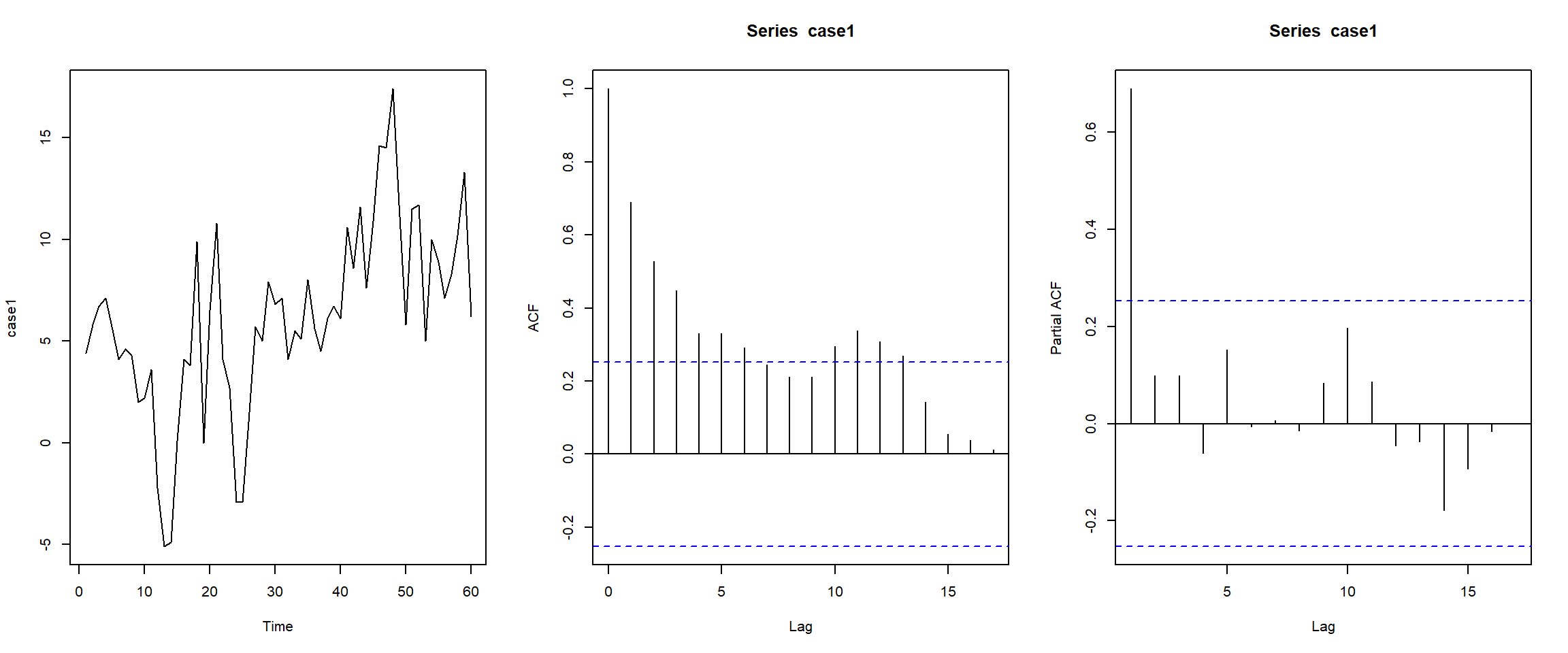1. estimation of model parameters
2. diagnostic checking: does the model fit the data? If yes, go ahead with forecast; if no, go back to (1).

In this course, we will follow this procedure as much as we can.

Other thinkings:

“Machine learning has become alchemy.” | Ali Rahimi, Google. https://www.youtube.com/watch?v=x7psGHgatGM “Without deep understanding of the basic tools needed to build and train new algorithms, he says, researchers creating AIs resort to hearsay, like medieval alchemists.” Cite

ARIMA Models

First let $$\tilde{Z}_t=Z_t-\mu$$, i.e., centered random variable. Here is a list of all ARIMA models.

Autoregressive models (AR) \begin{align*} AR(1): \tilde{Z}_t &= \phi_1 \tilde{Z}_{t-1} + a_t\\ AR(2): \tilde{Z}_t &= \phi_1 \tilde{Z}_{t-1} + \phi_2 \tilde{Z}_{t-2} + a_t\\ &...\\ AR(p): \tilde{Z}_t &= \phi_1 \tilde{Z}_{t-1} + ... + \phi_p \tilde{Z}_{t-p} + a_t \end{align*}

where $$a_t$$ is white noise and is commonly referred to as “innovation” or “shock.”

Why Innovation? The predictable components of your time-series are removed, so the leftover seems the only unknown thing. So is was called “innovation”.

Moving average models (MA) \begin{align*} MA(1): \tilde{Z}_t &= a_t - \theta_1 a_{t-1}\\ MA(2): \tilde{Z}_t &= a_t - \theta_1 a_{t-1} - \theta_2 a_{t-2}\\ &...\\ MA(q): \tilde{Z}_t &= a_t - \theta_1 a_{t-1} - ... - \theta_q a_{t-q} \end{align*}

Autoregressive moving average models (ARMA) \begin{align*} ARMA(1,1): \tilde{Z}_t &= \phi_1 \tilde{Z}_{t-1} + a_t - \theta_1 a_{t-1}\\ &...\\ ARMA(p,q): \tilde{Z}_t &= \phi_1 \tilde{Z}_{t-1} + ... + \phi_p \tilde{Z}_{t-p} + a_t - \theta_1 a_{t-1} - ... - \theta_q a_{t-q} \end{align*}

For ARIMA, we will come back to it later.

Notations for AR, MA, ARMA models:

If we define
$\phi(B) = 1 - \phi_1 B - \phi_2 B^2 - ... -\phi_p B^p\\ \theta(B) = 1 - \theta_1 B - \theta_2 B^2 - ... -\theta_q B^q$ as two polynomials of order $$p$$ and $$q$$.

Then AR(p) can be written as \begin{align*} \phi(B)\tilde{Z}_t&=a_t\\ (1 - \phi_1 B - ... -\phi_p B^p)\tilde{Z}_t&=a_t\\ \tilde{Z}_t - \phi_1 \tilde{Z}_{t-1} - ... -\phi_p \tilde{Z}_{t-p}&=a_t\\ \tilde{Z}_t &= \phi_1 \tilde{Z}_{t-1} + ... +\phi_p \tilde{Z}_{t-p} +a_t\\ \end{align*}

Similarly, MA(q) can be written as \begin{align*} \tilde{Z}_t&= \theta(B)a_t\\ \tilde{Z}_t&= (1 - \theta_1 B - ... -\theta_q B^q)a_t\\ \tilde{Z}_t&= a_t - \theta_1 a_{t-1} - ... - \theta_q a_{t-q}\\ \end{align*}

For example, AR(1) is $$(1 - \phi_1 B)\tilde{Z}_t=a_t$$ and MA(1) is $$\tilde{Z}_t=(1 - \theta_1 B)a_t$$.

Lastly, ARMA(p,q) can be written as \begin{align*} \phi(B)\tilde{Z}_t&=\theta(B)a_t\\ (1 - \phi_1 B - ... -\phi_p B^p)\tilde{Z}_t&=(1 - \theta_1 B - ... -\theta_q B^q)a_t\\ \tilde{Z}_t - \phi_1 \tilde{Z}_{t-1} - ... -\phi_p \tilde{Z}_{t-p}&=a_t - \theta_1 a_{t-1} - ... - \theta_q a_{t-q}\\ \tilde{Z}_t &= \phi_1 \tilde{Z}_{t-1} + ... +\phi_p \tilde{Z}_{t-p} +a_t - \theta_1 a_{t-1} - ... - \theta_q a_{t-q}\\ \end{align*}

# 2 AR models

## 2.1 AR(1)

AR(1) can be written as any of the following forms. \begin{align*} \phi(B)\tilde{Z}_t&=a_t\\ (1 - \phi_1 B)\tilde{Z}_t&=a_t\\ \tilde{Z}_t &= \phi_1 \tilde{Z}_{t-1} + a_t\\ Z_t-\mu &= \phi_1 (Z_{t-1}-\mu) + a_t\\ Z_t &= c + \phi_1 Z_{t-1} + a_t\\ \end{align*} where $$c=\mu(1-\phi_1)$$.

Assumptions:

1. $$a_t\sim N(0, \sigma_a^2)$$, meaning that the errors are independently distributed as a white noise that has mean 0 and constant variance.
2. Properties of the errors $$a_t$$ are independent of $$\tilde{Z}_t$$.
3. The series $$\tilde{Z}_1$$, $$\tilde{Z}_2$$, … is (weakly) stationary. A requirement for a stationary AR(1) is that $$|\phi|<1$$. We’ll see why below.

Exercise:

1. Simulat an AR(1) process with $$\phi=0.8$$, $$t=1,\dots,5000$$ ($$Z_t = c + \phi Z_{t-1} + a_t$$). Plot the time series;
2. Write your own function to simulate this AR(1) process;
3. Check the mean, variance, and the correlation between observations 1 time periods apart.

Properties of the AR(1):

Formulas for the mean, variance, and ACF for a time series process with an AR(1) model follow.

• The (theoretical) mean of $$Z_t$$ is $E(Z_t)=\mu = \dfrac{c}{1-\phi_1}$

• The variance of $$\tilde{Z}_t$$ is $\text{Var}(\tilde{Z}_t) = \dfrac{\sigma^2_a}{1-\phi_1^2}$

• The correlation between observations $$k$$ time periods apart is $\rho_k = \phi^k_1$

This defines the theoretical ACF for a time series variable with an AR(1) model.

Note!

$$\phi_1$$ is the slope in the AR(1) model and we now see that it is also the lag 1 autocorrelation.

To ensure AR(1) is stationary (we mostly work with the concept of weakly stationary through the course), we need $$|\phi_1|<1$$.

To see why $$|\phi_1|<1$$ is sufficient for stationarity, we can rewrite the AR(1) as \begin{align*} \tilde{Z}_t &= \phi_1 \tilde{Z}_{t-1} + a_t\\ &= \phi_1 (\phi_1 \tilde{Z}_{t-2} + a_{t-1}) + a_t\\ &...\\ &= \phi_1^k \tilde{Z}_{t-k} + \phi_1^{k-1} a_{t-k+1} + ... \phi_1 a_{t-1} + a_t \end{align*}

Let $$k \to \infty$$, then since $$|\phi_1|<1$$, we have $$\phi_1^k \to 0$$, that is \begin{align*} \tilde{Z}_t &= \sum_{j=1}^{\infty} \phi_1^{j} a_{t-j} + a_t \end{align*} Therefore, $$\mathbb{E}Z_t=0$$, $$\text{Var}[Z_t]=\sum_{j=1}^{\infty} \phi_1^{2j} \sigma^2_a + \sigma^2_a$$, and $$\gamma_k$$ and so on.

A more general rule for ensuring stationarity is that the root of the polynomial $$(1-\phi_1B)=0$$ to be outside of the unit circle. \begin{align*} 1-\phi_1 B = 0 \Rightarrow B=\frac{1}{\phi_1} \end{align*} To let the root be outside of the unit circle, we have \begin{align*} |B|=\Big|\frac{1}{\phi_1}\Big| > 1 \quad \Rightarrow \quad |\phi_1|<1 \end{align*} That is, AR(1) is stationary if and only if $$|\phi_1|<1$$.

Remark: the polynomial $$\phi(B)=1-\phi_1B=0$$ is called the characteristic equation for this time series model. The root of the equation is $$B=1/\phi_1$$. Note that the stationary condition $$|\phi_1|<1$$ is equivalent to the condition that the roots of the characteristic equation $$\phi(B)=0$$ lie outside of the unit circle of the complex plane.

Remark: If $$\phi_1=1$$, the model becomes $\tilde{Z}_t = \tilde{Z}_{t-1} + a_t.$ This model is not stationary (verify it). This is called a random walk, commonly used to model stock prices and many other economic activities. Note that if we define $$W_t=\tilde{Z}_t - \tilde{Z}_{t-1}$$, i.e., 1st order difference of $$Z_t$$, then $$W_t=a_t$$ is stationary, in fact, it is a white noise. This means that by differencing the data from the nonstationary time series model, we can transform data to a stationary time series model. When $$|\phi_1|=1$$, we call this AR(1) homogeneous non-stationary.

Here, we simulate data according to AR(1) using different values of $$\phi_1=0.1,0.5,0.95,1$$. As we can see as long as $$|\phi_1|<1$$, the time series stays in certain range, i.e., constant variance, which indicates stationarity.

set.seed(1234)
n=2000
ts1=arima.sim(n = n, list(ar = 0.1,  ma = 0), sd = 1)
ts2=arima.sim(n = n, list(ar = 0.5,  ma = 0), sd = 1)
ts3=arima.sim(n = n, list(ar = 0.95,  ma = 0), sd = 1)
ts4=as.ts(cumsum(rnorm(n)))
ts.plot(ts4,ts3,ts2,ts1,col=c("black","red","blue","green"))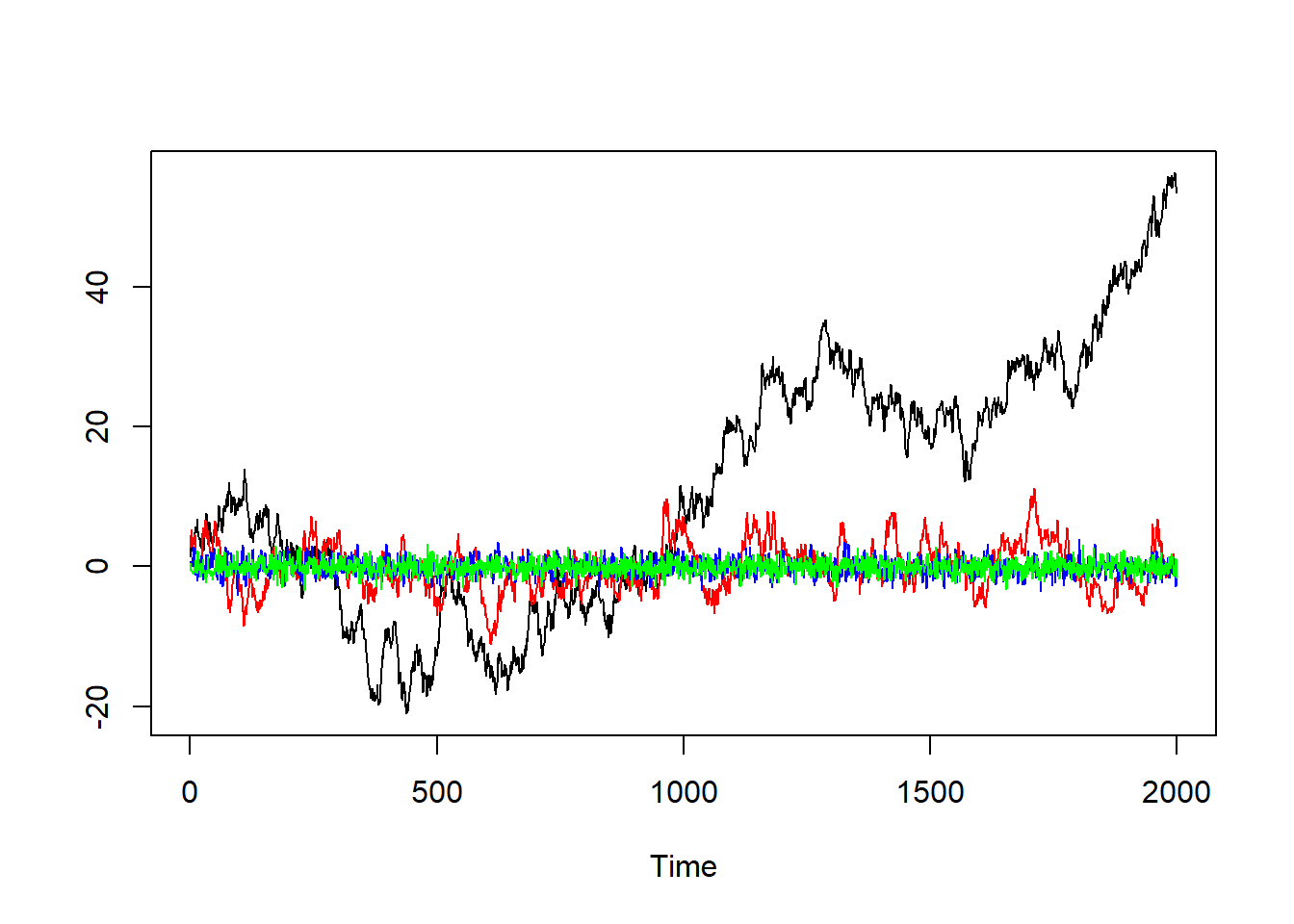When $$\phi_1=1$$, the time series explodes. In fact, we repeatedly generate from AR(1) with $$\phi_1=1$$, and the results are more obvious.

set.seed(1234)
MC=50
n=500
ts_mat=matrix(NA,n,MC)
for (it in 1:MC)
{
ts_mat[,it]=as.ts(cumsum(rnorm(n)))
}
ts.plot(ts_mat)
abline(h=0,col="blue")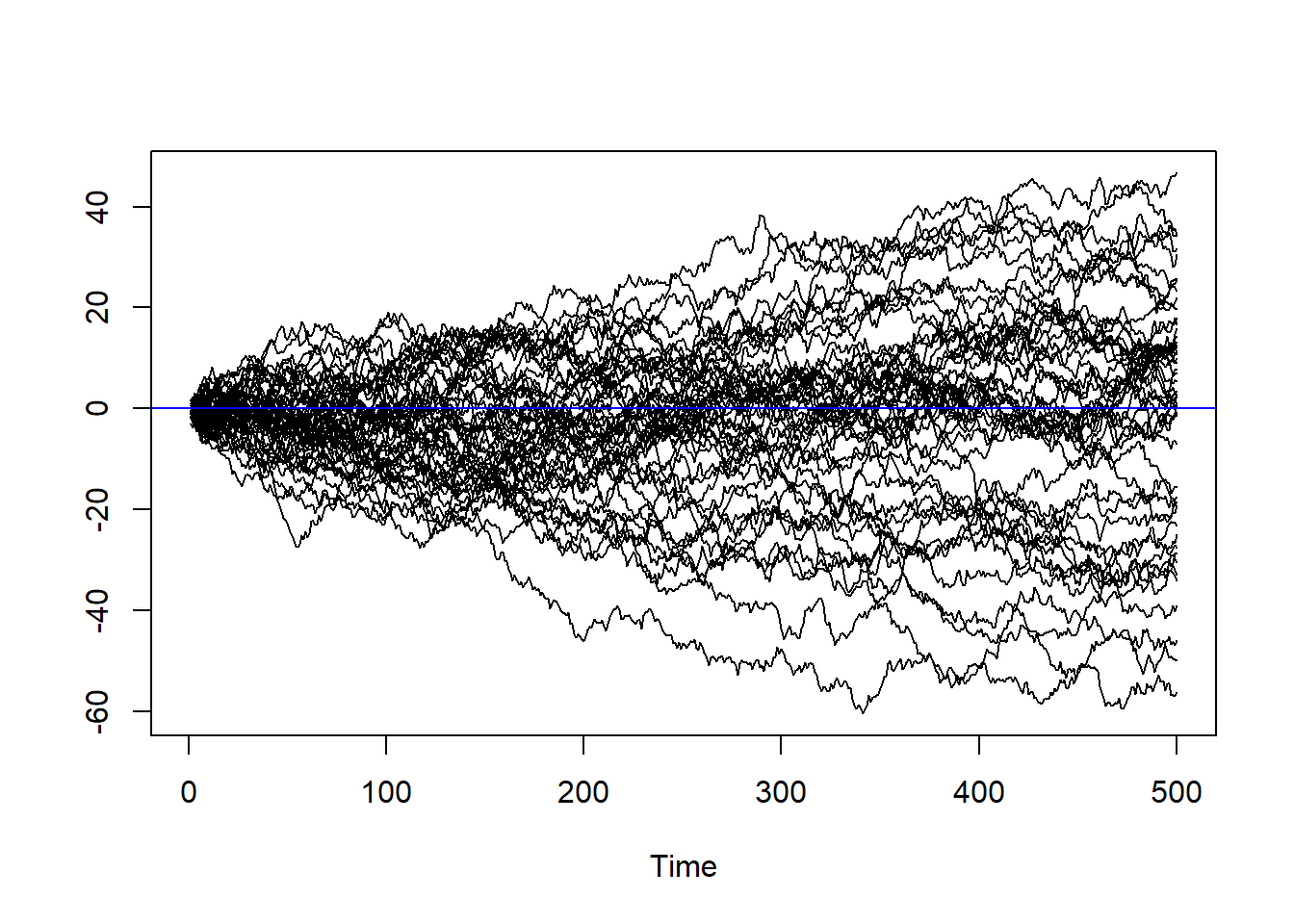Unit root: $$\phi_1=1$$ is called unit root because the root of $$\phi(B)$$ is on the unit circle. We need to be careful with unit root problem because it destroys the stationarity that we assume in the model. Often times, in real data that we work on, $$\phi_1$$ is not exactly 1 but $$\phi_1 \approx 1$$, this is called near unit root.

For the rest of this section, let’s assume $$|\phi_1|<1$$

Autocovariance of AR(1):

\begin{align*} \gamma_k &= \mathbb{E}[\tilde{Z}_t \tilde{Z}_{t-k}]= \mathbb{E}[\phi_1 \tilde{Z}_{t-1} \tilde{Z}_{t-k} + \tilde{Z}_{t-k} a_t]\\ &= \phi_1 \gamma_{k-1} + 0 = \phi_1 \phi_1 \gamma_{k-2}\\ &= \phi_1^k \gamma_{0} \end{align*} Note that $$\mathbb{E}[\tilde{Z}_{t-k} a_t]=0$$ because $$\tilde{Z}_{t-k}$$ and $$a_t$$ are independent, that is, $$a_t$$ happens after $$\tilde{Z}_{t-k}$$ which only depends on $$a_{t-k}, a_{t-k-1},...$$

Variance of AR(1)

Starting from the definition of variance, we have \begin{align*} \text{Var}[\tilde{Z}_t] &=\mathbb{E} [(Z_t-\mu)^2] \\ &=\mathbb{E} [\tilde{Z}_t^2]\\ &=\phi_1^2 \mathbb{E}[\tilde{Z}_{t-1}^2] + 2 \mathbb{E} [\tilde{Z}_{t-1} a_t] + \mathbb{E} [a_t^2]\\ &= \phi_1^2 \text{Var}[\tilde{Z}_{t-1}] + \sigma^2_a\\ &= \phi_1^2 \text{Var}[\tilde{Z}_t] + \sigma^2_a \end{align*} The last step is because of stationarity, i.e., variance unchanged. Therefore, we have \begin{align*} \text{Var}[\tilde{Z}_t] =\frac{\sigma^2_a}{1 - \phi_1^2} \end{align*}

It is easy to see that if $$\phi_1=1$$, the variance explodes.

Estimation of Autocovariance of AR(1):

ACF of AR(1): By the definition of ACF, we have ACF of AR(1) as \begin{align*} \rho_k=\frac{\gamma_k}{\gamma_0}=\begin{cases} 1, \quad k=0\\ \phi_1^{|k|}, \quad k \neq 0 \end{cases} \end{align*}

In practice, we never know the true autocovariance, and the true ACF $$\rho_k$$, so we have to estimate the ACF by sample ACF, $$\hat{\rho}_k$$.

$\hat{\rho}_k=\frac{\hat{\gamma}_k}{\hat{\gamma}_0}$

We need to estimate it by $\hat{\gamma}_0 = \frac{1}{n} \sum_{t=1}^{n} (z_t-\bar{z})^2$ $\hat{\gamma}_1 = \frac{1}{n-1} \sum_{t=2}^{n} (z_t-\bar{z})(z_{t-1}-\bar{z})$ $\hat{\gamma}_k = \frac{1}{n-k} \sum_{t=1+k}^{n} (z_t-\bar{z})(z_{t-k}-\bar{z})$ where $$\bar{z}=1/n \sum_{t=1}^{n} z_t$$ is the sample mean.

Here are some examples of AR(1) with their ACFs. We set $$\phi_1=0.5,-0.5$$

n=200000
ts1=arima.sim(n = n, list(ar = 0.5),  sd = 1)
ts2=arima.sim(n = n, list(ar = -0.5), sd = 1)
par(mfrow=c(2,3))
acf(ts1,ylim=c(-1,1),lag.max=15)
plot.ts(ts1[1:500])
acf(ts1[1:500],ylim=c(-1,1),lag.max=15)
acf(ts2,ylim=c(-1,1),lag.max=15)
plot.ts(ts2[1:500])
acf(ts2[1:500],ylim=c(-1,1),lag.max=15)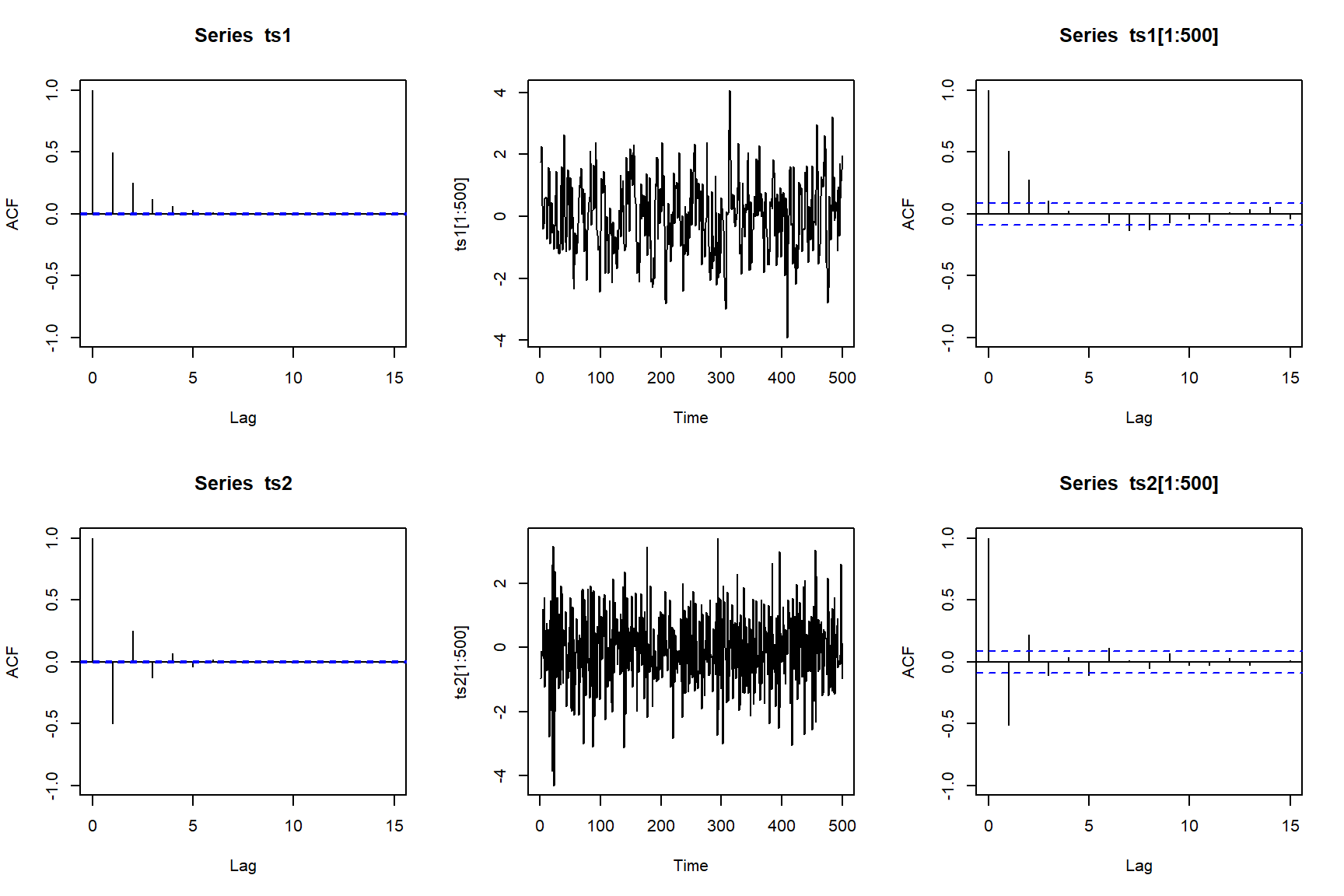Some extreme examples of AR(1). We set $$\phi_1=0.95,-0.95$$

n=200000
ts1=arima.sim(n = n, list(ar = 0.95),  sd = 1)
par(mfrow=c(2,3))
acf(ts1,ylim=c(-1,1),lag.max=60)
plot.ts(ts1[1:70])
abline(h=0)
acf(ts1[1:70],ylim=c(-1,1),lag.max=60)
ts2=arima.sim(n = n, list(ar = -0.95), sd = 1)
acf(ts2,ylim=c(-1,1),lag.max=60)
plot.ts(ts2[1:70])
abline(h=0)
acf(ts2[1:70],ylim=c(-1,1),lag.max=60)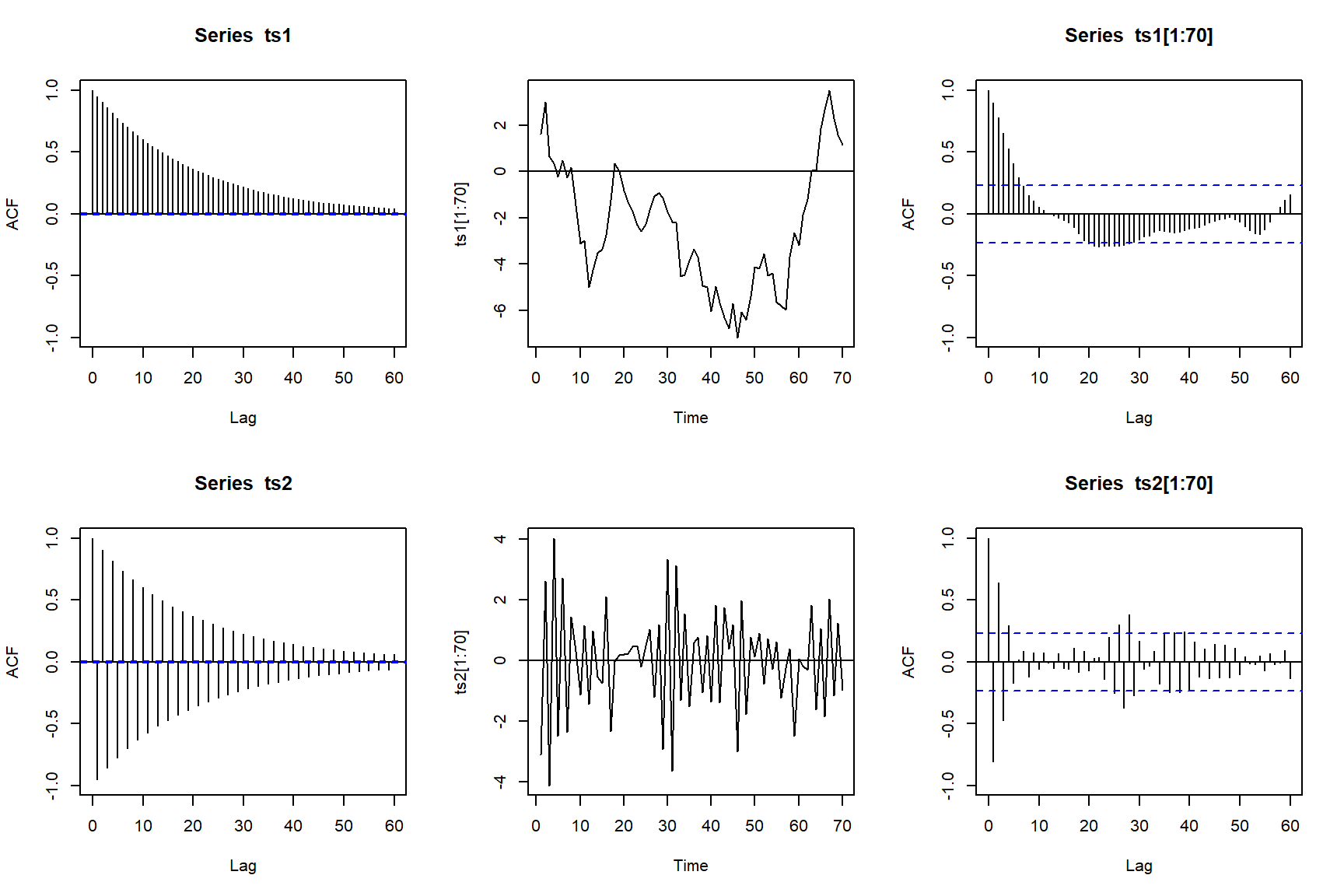The first example is called positive serial correlation in $$Z_t$$. The second is called negative serial correlation in $$Z_t$$.

PACF of AR(1)

Based on the relationship between ACF and PACF, we can derive the PACF of AR(1) as

\begin{align*} \phi_{kk}=\begin{cases} \phi_1=\rho_1, \quad k=1\\ 0, \quad \quad k \geq 1 \end{cases} \end{align*}

Here are some examples of AR(1) with their PACFs. We set $$\phi_1=0.5,-0.5$$

n=200000
ts1=arima.sim(n = n, list(ar = 0.5),  sd = 1)
ts2=arima.sim(n = n, list(ar = -0.5), sd = 1)
par(mfrow=c(2,3))
pacf(ts1,ylim=c(-1,1),lag.max=10)
plot.ts(ts1[1:300])
pacf(ts1[1:300],ylim=c(-1,1),lag.max=10)
pacf(ts2,ylim=c(-1,1),lag.max=10)
plot.ts(ts2[1:300])
pacf(ts2[1:300],ylim=c(-1,1),lag.max=10)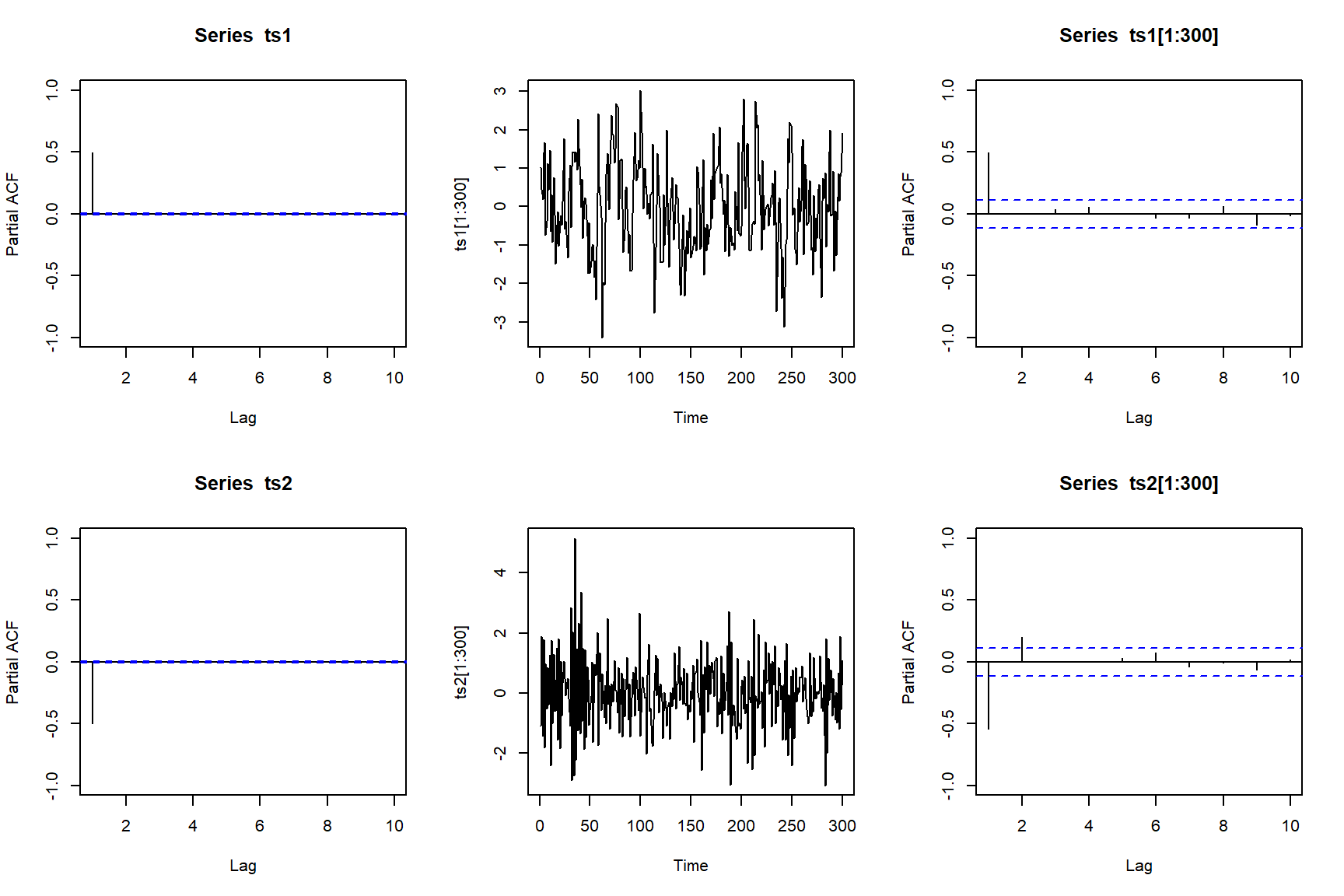## 2.2 AR(2)

AR(2) can be written as any of the following forms. \begin{align*} \phi(B)\tilde{Z}_t&=a_t\\ (1 - \phi_1 B - \phi_2 B^2)\tilde{Z}_t&=a_t\\ \tilde{Z}_t &= \phi_1 \tilde{Z}_{t-1} + \phi_2 \tilde{Z}_{t-2} + a_t\\ Z_t-\mu &= \phi_1 (Z_{t-1}-\mu) + \phi_2 (Z_{t-2}-\mu) + a_t\\ Z_t &= c + \phi_1 Z_{t-1} +\phi_2 Z_{t-2} + a_t\\ \end{align*} where $$c=\mu(1-\phi_1-\phi_2)$$.

To ensure AR(2) is stationary, we need the roots (i.e., two roots) of the polynomial $$(1-\phi_1B-\phi_2B^2)=0$$ to be outside of the unit circle.

Denote two roots as \begin{align*} R_1, R_2 = \frac{-\phi_1 \pm \sqrt{\phi_1^2 + 4 \phi_2}}{2\phi_2}\\ |R_1|>1, |R_2|>1 \Leftrightarrow \begin{cases} \phi_1+\phi_2<1\\ \phi_2-\phi_1<1\\ -1<\phi_2<1 \end{cases} \end{align*}

In other words, the pair $$(\phi_1, \phi_2)$$ has to be inside of the triangle below in order to have stationary AR(2).

plot(0,0,type="n",xlab="phi_1",ylab="phi_2",xlim=c(-3,3),ylim=c(-2,2))
abline(h=0,col="grey")
abline(v=0,col="grey")
lines(c(0,2),c(1,-1))
lines(c(0,-2),c(1,-1))
lines(c(-2,2),c(-1,-1))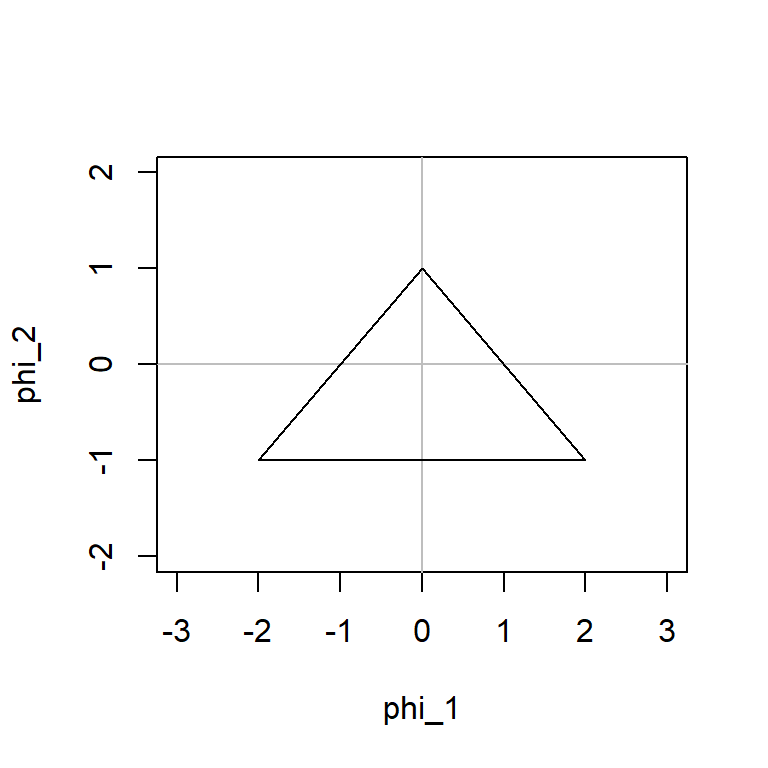Autocovariance of AR(2) \begin{align*} \mathbb{E}[\tilde{Z}_t \tilde{Z}_{t-k}] &= \mathbb{E}[\phi_1 \tilde{Z}_{t-1} \tilde{Z}_{t-k} + \phi_2 \tilde{Z}_{t-2} \tilde{Z}_{t-k} + \tilde{Z}_{t-k} a_t]\\ \gamma_k &= \phi_1 \gamma_{k-1} + \phi_2 \gamma_{k-2}\\ \rho_k &= \phi_1 \rho_{k-1} + \phi_2 \rho_{k-2} \end{align*} This is called Yule-Walker equation (explained later).

Let $$k=1,2$$, we have the following (note $$\rho_0=1$$, $$\rho_{-1}=\rho_1$$, and $$\rho_{-2}=\rho_2$$… )

\begin{align*} \begin{array} {r} \rho_1 = \phi_1 + \phi_2 \rho_1\\ \rho_2 = \phi_1 \rho_1 + \phi_2 \end{array} \Leftrightarrow \begin{cases} \rho_1 = \frac{\phi_1}{1- \phi_2}\\ \rho_2 = \frac{\phi_1^2}{1- \phi_2} + \phi_2 \end{cases} \Leftrightarrow \begin{cases} \phi_1 = \frac{\rho_1 (1-\rho_2)}{1- \rho_1^2}\\ \phi_2 = \frac{\rho_2-\rho_1^2}{1- \rho_1^2} \end{cases} \end{align*}

For $$k>2$$, we have \begin{align*} \rho_k = \phi_1 \rho_{k-1} + \phi_2 \rho_{k-2} \end{align*}

Variance of AR(2)

Set $$k=0$$, we have \begin{align*} \mathbb{E}[\tilde{Z}_t \tilde{Z}_{t}] &= \mathbb{E}[\phi_1 \tilde{Z}_{t-1} \tilde{Z}_{t} + \phi_2 \tilde{Z}_{t-2} \tilde{Z}_{t} + \tilde{Z}_{t} a_t]\\ \gamma_0 &= \phi_1 \gamma_1 + \phi_2 \gamma_2 + \sigma^2_a\\ \gamma_0 &= \phi_1 \rho_1 \gamma_0 + \phi_2 \rho_2 \gamma_0 + \sigma^2_a\\ \end{align*}

we can obtain the variance of AR(2) as \begin{align*} \text{Var}(Z_t)=\gamma_0=\frac{\sigma^2_a}{1-\rho_1 \phi_1 - \rho_2 \phi_2} \end{align*}

ACF of AR(2)

\begin{align*} \rho_k=\begin{cases} 1, \qquad \quad \quad k=0\\ \frac{\phi_1}{1- \phi_2}, \quad \quad \quad \quad k=1\\ \frac{\phi_1^2}{1- \phi_2} + \phi_2, \quad \quad k=2\\ \phi_1 \rho_{k-1} + \phi_2 \rho_{k-2}, \quad k > 2 \end{cases} \end{align*}

Here are some examples of AR(2) with their ACFs. We set $$\phi_1=+/-0.3$$,$$\phi_2=+/-0.5$$.

n=200000
ts1=arima.sim(n = n, list(ar = c(0.3, 0.5)), sd = 1)
ts2=arima.sim(n = n, list(ar = c(0.3,-0.5)), sd = 1)
ts3=arima.sim(n = n, list(ar = c(-0.3,0.5)), sd = 1)
ts4=arima.sim(n = n, list(ar = c(-0.3,-0.5)),sd = 1)
par(mfrow=c(2,2))
acf(ts1,ylim=c(-1,1),lag.max=25)
acf(ts2,ylim=c(-1,1),lag.max=25)
acf(ts3,ylim=c(-1,1),lag.max=25)
acf(ts4,ylim=c(-1,1),lag.max=25)